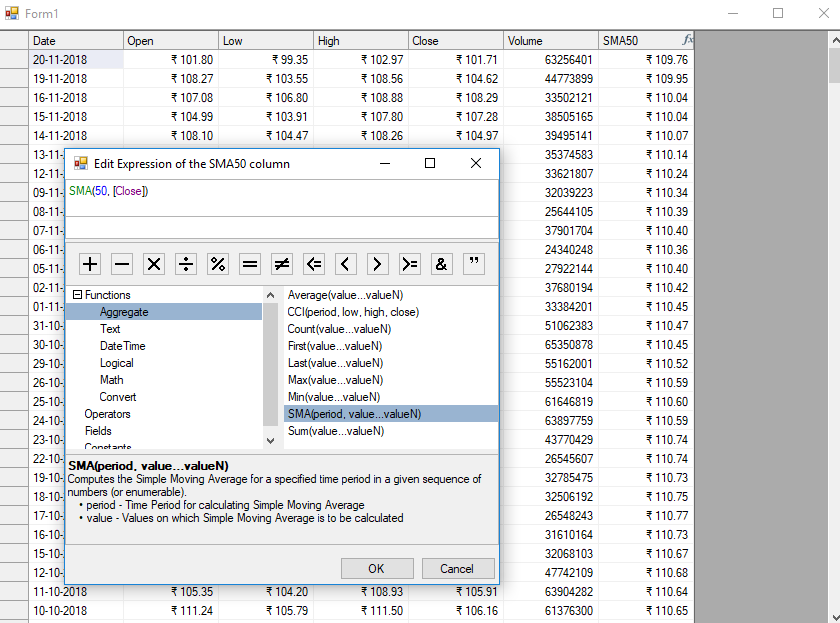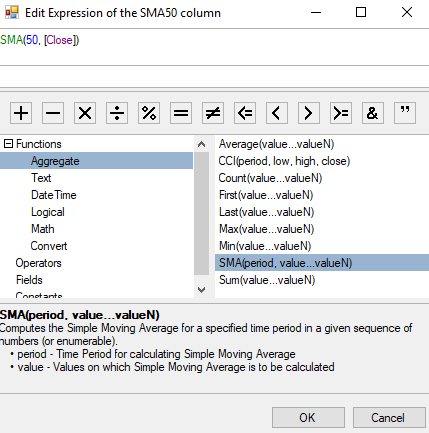﻿ 5 Steps to Generate Custom .NET Functions Using Expression Editor | ComponentOne# 5 Steps to Generate Custom .NET Functions Using Expression Editor

When using the ExpressionEditor, a user may need to evaluate expressions. For example, in a finance application, you may need to create a metric, like a simple moving average (SMA), to study the daily changes in stock price to see a trend. Using the SMA metric helps to view and understand an aggregated trend. Using an expression editor, we can also modify the expression to see trends with different time periods.

Overall, using a custom function can help make sense of the available raw data.

The SMA is only one example, we can add many more complex functions. The sample attached to this blog also shows another trend function (commodity channel index).

C1ExpressionEditor is a standalone control that allows users to edit and evaluate expressions. While it has been possible to use simple expressions and built-in functions, it is also possible to add custom functions to the Expression Editor and leverage the same editing capabilities. With custom functions, you can add your own logic to evaluate an expression according to your application's requirements.

In this blog, we'll see how we can add our own custom functions to an Expression Editor and use it with C1FlexGrid. We'll create a simple moving average function that is commonly used in financial applications.

#### Here is the .NET expression output for our application:## Generating the Grid in Our .NET Application

To start, we'll load some financial data in our grid and add an unbound column to the same. This unbound column would be used to set up the expression editor containing our SMA function.

``````//Add unbound column
column.Name = "SMA50";
column.Caption = "SMA50";
column.AllowExpressionEditing = true;
column.DataType = typeof(double);
column.Format = "C2";
column.AllowEditing = false;
``````

### Setting up the ExpressionEditor

Next, we need an instance of C1ExpressionEditor where we'll add our custom functions. We use ExpressionEditorCollection, associate it with the grid, and add an ExpressionEditor to this collection.

``````//
Associate an ExpressionEditorCollection with the grid
_editorCollection = new
ExpressionEditorCollection(); _editorCollection.SetExpressionEditorCollection(_flexGrid, _editorCollection);
//
Add editor for SMA50 column in ExpressionEditorCollection
``````

### Setting up the ExpressionItem

An ExpressionItem is the atomic unit of an Expression. It can be a constant, field, operator, or a function. After setting up the expression editor, we now need to set up an ExpressionItem for our ExpressionEditor. It will help us define the syntax for our custom function, the arguments it will take, its description, and the ItemType of our custom function.

Since we're creating a function, we'll use an ItemType of Aggregate function here.

``````//Define ExpressionItem
var expressionItem = new ExpressionItem("SMA", "SMA()", ItemType.AggregateFuncs);
{
typeof(int)
}){
Description = "Time Period for calculating Simple Moving Average"
});
{
typeof(byte), typeof(short), typeof(int), typeof(long), typeof(double), typeof(decimal)
}){
Description = "Values on which Simple Moving Average is to be calculated"
});

expressionItem.IsMultiArguments = true;
expressionItem.Description = "Computes the Simple Moving Average for a specified time period in a given sequence of numbers (or enumerable).";
``````

### Defining the Evaluation Function

After the ExpressionItem has been defined we will write down the evaluation logic that would be calculating the SMAs. The ExpressionEditor would delegate to this function to evaluate the SMA expressions and display the results that it would return.

The evaluation function must have a List`<object>` argument and should return an Object. A function can take two types of arguments: an exact value (represented by a literal), and a column name enclosed in square-brackets ([]).

If ExpressionItem's ItemType is Aggregate, and it only contains single column-based argument, then List `<object>` will contain all the values of that column. In this case, the ExpressionEditor would evaluate the function once for all the values of that column. However, if the arguments passed are a mix of literal and column-based arguments, then the List `<object>` will contain the literal value and the value of the column's cell for which the function is being evaluated. The ExpressionEditor in this case would start evaluating each row in the order they are present in the DataSource.

``````private object SMA(List<object> values)
{
string colName = GetColumnName(_editor.Expression);
DataRow dataRow = null;

List<double> list = new List<double>();
int period = Convert.ToInt32(values);

if (_editorCollection.DataSource is DataTable)
{
dataRow = _editor.ItemContext as DataRow;
if (dataRow == null)
{
return null;
}

DataTable table = _editorCollection.DataSource as DataTable;

for (int rowIndex = table.Rows.IndexOf(dataRow); rowIndex < table.Rows.Count; ++rowIndex)
{
}
}

return CalculateSMA(period, list);
}
``````
``````private double? CalculateSMA(int period, List<double> values)
{
if (values == null || period > values.Count)
{
return null;
}
return values.Take(period).Average();
}
``````

Once the evaluation logic has been defined, we use the 'AddFunction' method of the expression editor to add the ExpressionItem that was defined earlier as well as this evaluation logic.

``````// Add the ExpressionItem and the SMA Custom Function to the editor
``````

That's it. Now we can use SMA function in the 'SMA50' column.## Additional Functions for the .NET ExpressionEditor

We can add any custom function to ExpressionEditor, from a simple function like 'Rank' for calculating an item's position in an ordered sequence of values to a complex function which can transform the actual data to something that is more relevant to a user.

Maybe you can localize your data values (and not just UI string) by creating a custom function that converts your database values into units preferred by your user.